# Maximally-flat unequal Wilkinson

Click here to go to our main page on Wilkinson power splitters

Click here to go to our main page on unequal Wilkinson power splitters

Click here to go to our page on unequal Wilkinsons, the rest of the story

On this page we analyze three different solutions to an unequal-split Wilkinson, with P3/P2 power ratio of 0.5 (-3.01 dB difference, upper arm favored). Below is a schematic that defines the nomenclature we are using.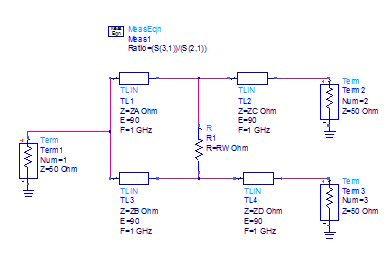### Parad and Moynihan's Split Tee solution

Parad and Moynihan wrote the classic paper on unequal-split Wilkinson, titled "Split Tee Power Divider", way back in 1965. The Parad and Moynihan solution impedance values for P3/P2=0.5 are:

ZA=51.5 ohms
ZB=103 ohms
ZC=42.05 ohms
ZD=59.47 ohms
RW=106.09 ohms

Below we have plotted the S-parameter response for such a circuit if it was centered at 1 GHz.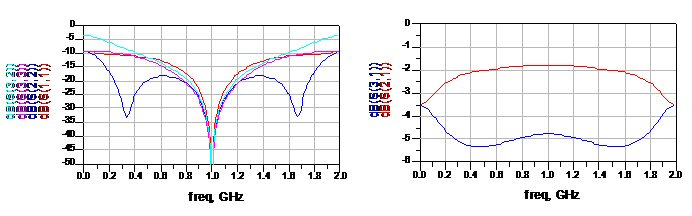Response using Parad and Moynihan equations

Below we used some equations to plot the coupling ratio, on the left is power (for which it is necessary to square the S-parameter ratio of S31/S21 as S-parameters are in voltage), and on the right it is plotted in decibels.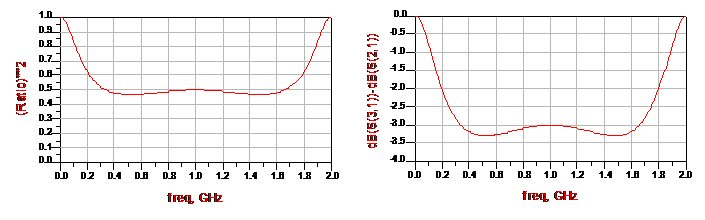Coupling ratio (power on left, decibels on right)

### Maximally-flat impedance transformer in weaker arm

The max flat transformer is an interesting piece of math. Learn about it on this page. Of course, we used our free transformer download to come up with the values for ZB and ZD (the weaker arm). The values for ZA and ZC follow from the equations we posted here.

The max flat transformer in the weaker arm transforms 50 to 150 ohms through ZB and ZD. The values are:

ZA=57 ohms
ZB=114 ohms
ZC=46.54 ohms
ZD=65.82 ohms
RW=129.96 ohms

Below are the responses.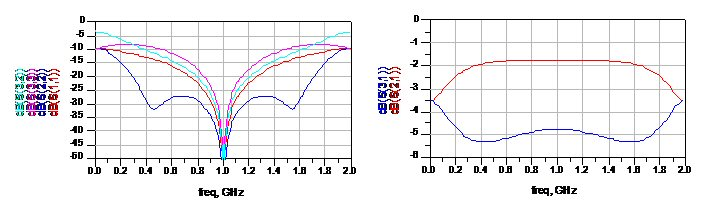Response using max flat transformer in weaker arm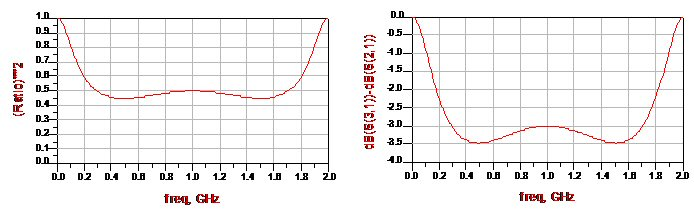Coupling ratio using max flat transformer in weaker arm (power on left, decibels on right)

### Maximally-flat impedance transformer in stronger arm

Next we applied a max flat transformer in the stronger arm (in this case it transforms 50 to 75 ohms through ZA and ZC). The values are:

ZA=67.8 ohms
ZB=135.6 ohms
ZC=55.34 ohms
ZD=78.29 ohms
RW=183.87 ohms

Below the responses are plotted.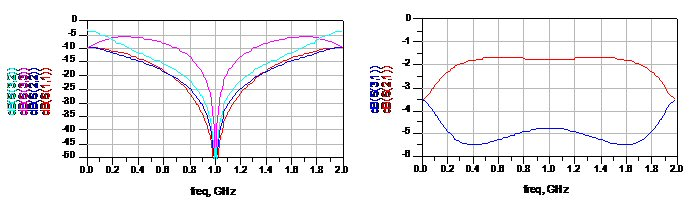Response using max flat transformer in stronger armCoupling ratio using max flat transformer in stronger (power on left, decibels on right)

### Conclusion

If you compare the bandwidth of the splitters, it seems the the original Split Tee equations hold up well in this one example. They provide the most bandwidth for coupling of the intended -3 dB. In this case, they provided a flatter response than the using the max flat transformation, who would have predicted that? Of course, we were using the max flat transformer in a way that it was not intended.

Of the two max-flat solutions, the one with the transformer in the weaker arm is preferred.

Now that we have thoroughly beat this topic to death, maybe we can move on to something fun like electronic warfare!

Author : Unknown Editor